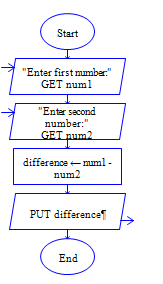# Python Subtraction

Python program to get two integer numbers, subtract both the integers and display the difference

Sample Input 1:

6 5

Sample Output 1:

1

Sample Input 2:

65 4

Sample Output 2:

61

#### Flow Chart DesignStrongly recommended to Solve it on your own, Don't directly go to the solution given below.

#### Program or Solution

``` num1=int(input("Enter First number:")) num2=int(input("Enter second number:")) diff=num1-num2 print(diff) ```

#### Program Explanation

Read two inputs using input() method.

Convert it into integer by int() method.

Subtract it using - operator.